Students get through the TN Board 12th Chemistry Important Questions Chapter 8 Ionic Equilibrium which is useful for their exam preparation.

## TN State Board 12th Chemistry Important Questions Chapter 8 Ionic Equilibrium

Question 1.
State the formula and the name of the conjugate base of each of the following acids.
(a) H3O+,
(b) HSO4,
(c) HF,
(d) NH4+,
(e) CH3NH3+,
(f) CH3PO4,
(g) CH3COOH,
(h) H2PO4,
(i) HS,
(j) HCO3.
(a) H2O – water
(b) SO4-2 – sulphate ion
(c) F – fluoride ion
(d) NH3 – ammonia
(e) CH3NH2 – methyl amine
(f) H2PO4 – dihydrogen phosphate ion
(g) CH3COO – acetate ion
(h) HPO4-2 – hydrogen phosphate ion
(i) S-2 – sulphide ion
(j) CO3-2 – carbonate ion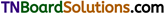Question 2.
State the formula and name of the conjugate acid of each of the following:
(a) OH,
(b) CH3COOH,
(c) HPO4-2,
(d) CO3-2,
(e) NH3,
(f) CH3NH2,
(g) HS,
(h) CH3COO
(i) H2PO4,
(j) Cl.
(a) H3O – water
(b) CH3COOH2+ – protonated acetic acid
(c) H2PO4 – dihydrogen phosphate ion
(d) HCO3 – bicarbonate ion
(e) NH4+ – ammonium ion
(f) CH3NH3+ – protonated methyl amine
(g) H2S – hydrogen sulphide
(h) CH3COO – acetic acid
(i) H3PO4 – phosphoric acid
(j) HCl – hydrochloric acid

Question 3.
Which of the following behave both as Bronsted acids as well as Bronsted bases?
NH3, HSO4, H2SO4, HCO3; H3PO4, HS, H2O.
NH3, HSO4 , HCO3 ; HS and H2O behave both as Bronsted acids and Bronsted bases.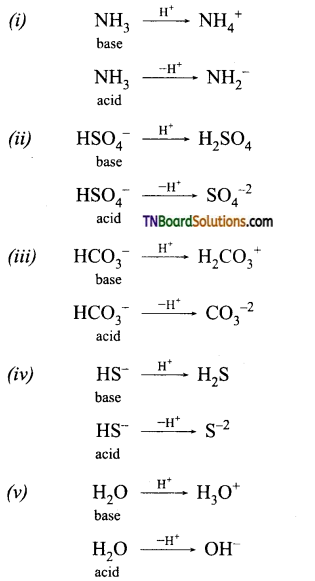Question 4.
Classify Lewis acids and bases from the following:
H3O+, CH3NH2, BF3, OH, Cu2+, S2-, CH3COO.
Lewis acids: H3O+, BF3, Cu2+.
Lewis bases: CH3NH2, OH, S2-, CH3COO.Question 5.
Classify the following species into Lewis acids and Lewis bases and show how these act as Lewis acid/base.
(a) OH, (b) F, (c) H+, (d) BCl3
(a) OH is a Lewis base because it acts as an electron-pair donor.
(b) F is also a Lewis base.
(c) H+ is a Lewis acid because it acts as an electron pair acceptor.
(d) BCl3 is an electron-deficient compound electron pair acceptor, hence Lewis acid.

Question 6.
Explain how the [H+] concentration or [OH] concentration, decides a solution as acidic, neutral, or alkaline.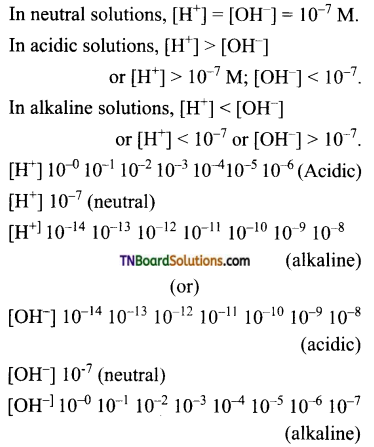Question 7.
Calculate the hydrogen and hydroxyl ion concentrations in (i) 0.01M HNO3, (ii) 0.001M KOH solution at 298 K.
(i) HNO3 is a strong acid. It ionizes as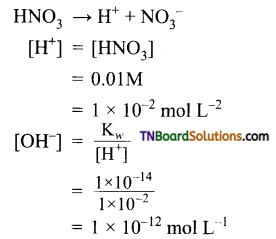(ii) KOH is a strong base. It ionizes as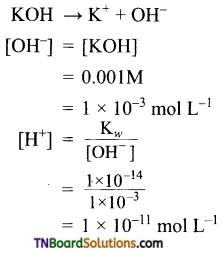Question 8.
Assuming complete dissociation, calculate the pH of the following solutions:
(i) 0.003M HCl,
(ii) 0.005M NaOH,
(iii) 0.002M HBr,
(iv) 0.002M KOH.
(i) HCl → H+ + Cl
[H+] = [HCl] = 0.003M
pH = – log [H+]
= – log [0.003] = 2.52(ii) NaOH → Na+ + OH
[OH] = [NaOH] = 0.005M
pOH = – log[OH]
= – log [0.005] = 2.301
pH = 14 – pOH
= 14 – 2.301 = 11.699

(iii) HBr → H+ + Br
[H+] = [HBr]
= 0.002M
pH = – log [H+] (or)
pH = – log [0.002] = 2.6989

(iv) KOH → K+ + OH
[OH] = [KOH]
= 0.002M
pOH = – log [OH ]
pH = – log (0.002) = 2.6929
pH = 14 – pOH (or)
= 14 – 2.6929 = 11.3011

Question 9.
Calculate the pH of the resulting mixtures: 10 ml of 0.02M Ca(OH)2 + 25 ml of 0.1M HCl.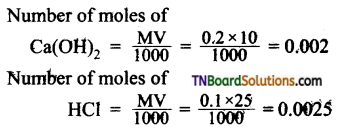Number of moles of OH ion = 2 x 0.002 = 0.004
Number of moles of H+ ion = 0.0025
Number of moles of OH remaining after neutralisation = 0.004 – 0.0023 = 0.0015
Molarity of OH ions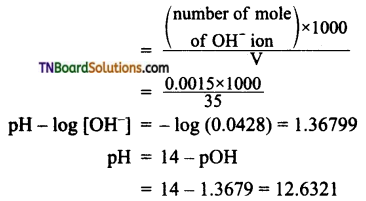Question 10.
The concentration of H+ ions in 0.1 M solution of a weak acid is 1.0 x 10-5 mol L-1. Calculate the dissociation constant of the acid.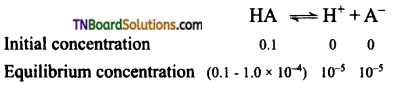[HA] can be taken as 0.1 as 1 x 10-5 is very small. Hence,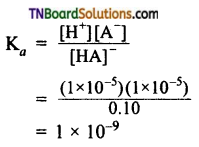Question 11.
Find whether the resulting solution is acidic, neutral, or basic when
(i) a strong acid is mixed with a strong base
(ii) a strong acid is mixed with a weak base
(iii) a weak acid is mixed with a strong base
(iv) a weak acid is mixed with a weak base.
(i) A strong acid and a strong base give a neutral solution because both are completely ionized and the reaction goes on completion.
eg: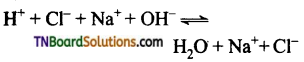(ii) A strong acid and a weak base give an acidic solution, as the weak acid is hot completely ionized. The reaction does not go for completion and there is an excess of hydrogen ions in the solution.
eg: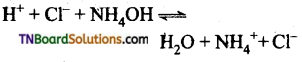The solution is therefore is acidic.
(iii) A weak acid and a strong base give a basic solution, as the weak acid is not completely ionized. The reaction does not go for completion and there is an excess of hydroxyl ions in the solution.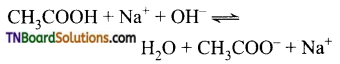Hence the solution is basic.
(iv) A weak acid and a weak base give an acidic, neutral, or basic depending on the relative strength of acid or base. If both weak acid and weak base have equal strength, the resulting solution is neutral.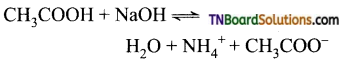Question 12.
State Ostwald’s dilution law.
When dilution increases, the degree of dissociation of weak electrolytes also increases.
The mathematical form is
α = $$\sqrt{\frac{\mathrm{K}_{a}}{\mathrm{C}}}$$
where ‘α’ is the degree of dissociation, Ka is the dissociation constant of the acid and ‘C’ is its concentration.

Question 13.
Explain the term ‘common ion effect’ with an example.
The suppression of the dissociation of a weak electrolyte by the addition of an ion in common with that of the weak electrolyte is known as the common ion effect.
Consider the dissociation of acetic acid in the presence of sodium acetate or HCl.
In the absence of any added salt, the dissociation of acetic acid is represented as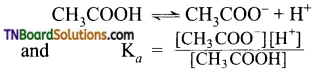The addition of acetate ions in the form of sodium acetate increases the concentration of acetate ions. This increases the value of Ka. But Ka is a constant at a given temperature. Thus to maintain the Ka value constant, H+ ions combine with acetate ion to form undissociated acetic acid. i.e., The degree of dissociation of acetic acid in the presence of acetate ion is less than in the absence of sodium acetate. The same happens in the presence of HCl.Question 14.
What are buffer solutions? Give example.

1. Buffer solutions resist changes in pH by the addition of small quantities of an acid or a base. This ability is known as buffer action.
2. Buffer solutions consist of a mixture of a weak acid and a salt of a weak acid and a strong base.
eg: CH3COOH + CH3COONa. This type of buffer is known as an acid buffer.
3. Buffer solution which consists of a mixture of a weak base and a salt of a weak base and strong acid is known as a basic buffer.
eg: NH4OH and NH4Cl.

Question 15.
Give the characteristics of a buffer solution.

1. It should have a definite pH. i.e., it has reserve acidity or alkalinity.
2. Its pH does not change on standing.
3. Its pH does not change on dilution.
4. Its pH is only slightly changed by the addition of a small quantity of acid or base.

Question 16.
Give examples of acidic and basic buffers.
Acidic buffer:

1. CH3COOH + CH3COONa
2. Boric acid and borax.
3. Pthalic acid and potassium acid pthalate.

Basic buffer:

1. NH4OH + NH4Cl
2. Glycine and glycine hydrochloride.
3. Aniline and aniline hydrochloride.

Question 17.
Explain buffer action with a suitable example.
The buffer action in a solution containing CH3COOH and CH3COONa is explained as follows:
The dissociation of the buffer components occurs as below.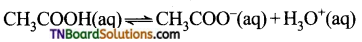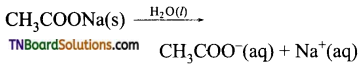If an acid is added to this mixture, it will be consumed by the conjugate base CH3COO to form the undissociated weak acid i.e., the increase in the concentration of H+ does not reduce the pH significantly.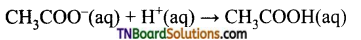If a base is added, it will be neutralized by H3O+, and the acetic acid is dissociated to maintain the equilibrium. Hence the pH is not significantly altered.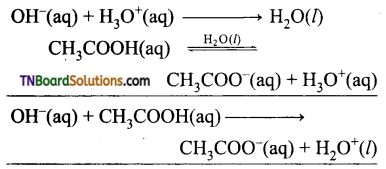Question 18.
Explain the terms ‘buffer capacity” and ‘buffer index”.
The buffer capacity is the ability of the buffer to resist changes in pH by the addition of small quantities of acid or base. It is measured in terms of buffer capacity. The quantitative measure of buffer capacity is the buffer index. It is defined as the number of equivalents of acid or base to one liter of the buffer solution to change its pH by unity.
β = $$\frac{d \mathrm{~B}}{d(\mathrm{pH})}$$
where P = buffer index, dB = number of gram equivalent of acid/base added to one liter of buffer solution, d(pH) = The change in pH after the addition of an acid or base.Question 19.
Derive Henderson equation for the determination of pH of an acid buffer.
An acid buffer consists of a mixture of a weak acid and salt with strong acid. The ionization of weak acid, HA is given by
HA ⇌ H+ + A
The dissociation constant of the weak acid is given by,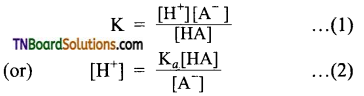It can be assumed that the concentration of [A] ions from the complete ionization of the salt compared to the ionization of the weak acid. Hence, [A] concentration may be considered as the concentration of the salt.
So,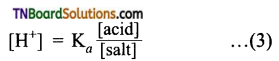Taking logarithms on both sides and reversing the sign,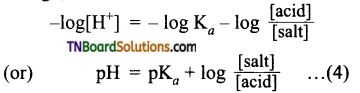This is known as Henderson’s equation.

Question 20.
Write Henderson’s equation for a basic buffer.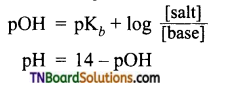Question 21.
Suppose it is required to make a buffer solution of pH = 4. Using acetic acid and sodium acetate. How much sodium acetate to be added to 1 liter of N/10 acetic acid? The dissociation constant of acetic acid is 1.8 × 10-5.
Applying Henderson’s equation,
The molecular mass of CH3COONa = 82
Amount of salt = 0.018 × 82 = 1.476 g.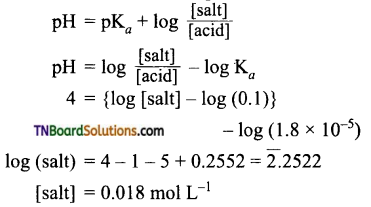Question 22.
Calculate the pH of a buffer solution containing 0.15 moles of NH4OH and 0.25 moles of NH4Cl. (Kb for NH4OH = 1.8 × 10-5)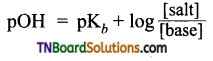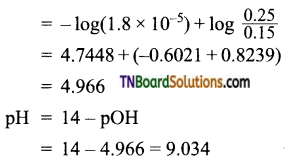Question 23.
Calculate the pH of 0.1M ammonia solution. Calculate the pH after 50 ml of this solution is treated with 25 ml of 0.1M HCl. The dissociation constant of ammonia, Kb = 1.77 × 10-5.
For NH4OH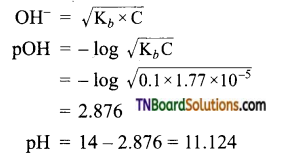When NH3 is added, neutralization occurs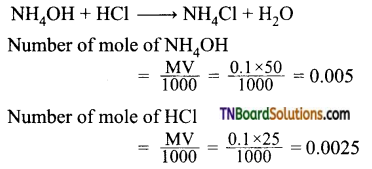(MNH4Cl) number of mole of NH4Cl = 0.0025
(MNH4OH) Remaining mole of NH4OH = 0.005 – 0.0025 = 0.0025
According to Henderson’s equation,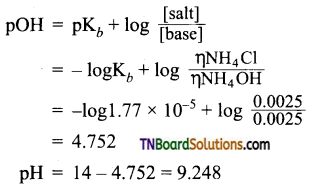Question 24.
Define hydrolysis.
The reaction between cation or anion of the salt with water to produce the acid and base from which the salt formed is known as hydrolysis.
salt + H2O = acid + baseQuestion 25.
Define the term degree of hydrolysis.
It is the fraction of one mole of the salt that undergoes hydrolysis is known as the degree of hydrolysis.

Question 26.
Define the term hydrolysis constant (Kh), with an example.
The equilibrium constant for the reaction, salt + H2O ⇌ acid + base, is known as the hydrolysis constant (Kh). Consider the hydrolysis of acetate ion.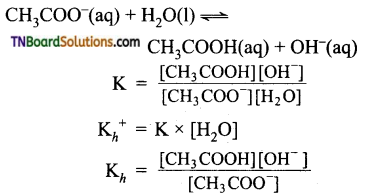Question 27.
The pKa of acetic acid and pKb of ammonium hydroxide are 4.76 and 4.75 respectively. Calculate the pH of the ammonium acetate solution.
Ammonium acetate is the salt of a weak acid and weak base.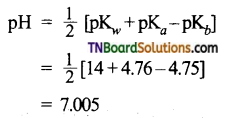Question 28.
The ionization constant of nitrous acid is 4.5 × 10-4. Calculate the pH of 0.04 M sodium nitrite solution and its degree of hydrolysis.
Sodium nitrite is a salt of a weak acid and a strong base.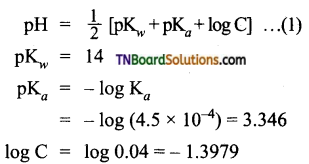Substituting these values in equation (1)
pH = $$\frac{1}{2}$$ [14 + 3.346 – 1.3979]
= 7.974
Degree of hydrolysis (h)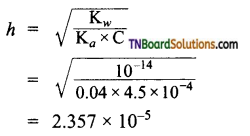Question 29.
Calculate the degree of hydrolysis and pH of a 0.1M sodium acetate solution. The hydrolysis constant of sodium acetate is 5.6 × 10-10.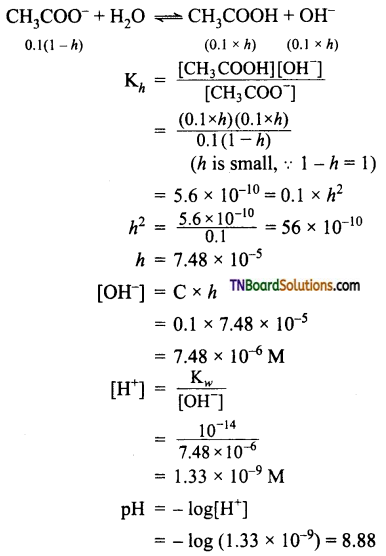Question 30.
Calculate the pH of an aqueous solution of 1.0M ammonium formate assuming complete dissociation. pKa for formic acid is 3.8 and pKb of ammonia = 4.8.
Ammonium formate is a salt of a weak acid and weak base.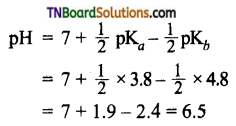Question 31.
A 0.02 M solution of pyridinium hydrochloride has pH = 3.44. Calculate the ionization constant of pyridine.
Pyridinium hydrochloride is a salt of a weak base and strong acid.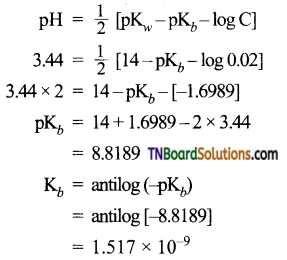Question 32.
Define solubility product.
It is defined as the product of the concentration of ions in a saturated solution of an electrolyte at a given temperature.

Question 33.
Give expressions for the solubility product of the following: (i) BaSO4, (ii) H2S, (iii) Sb2S3, (iv) AlI3.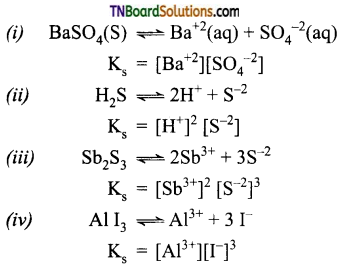Question 34.
Mention the criteria of precipitation of an electrolyte.
The ionic product should exceed the value of the solubility product, for precipitation to occur.

Question 35.
How will you decide whether a solution is saturated or unsaturated, from the ionic product values?

1. When the ionic product is less than the solubility product, the solution is unsaturated.
2. When an ionic product is equal to the solubility product, the solution is saturated.

Question 36.
Obtain a relationship between solubility and solubility product of the following: (i) AgCl, (ii) Ag2CO3, (iii) CaF2, (iv) Cr(OH)3.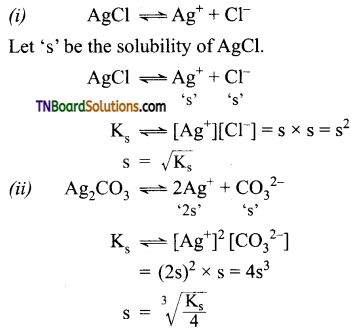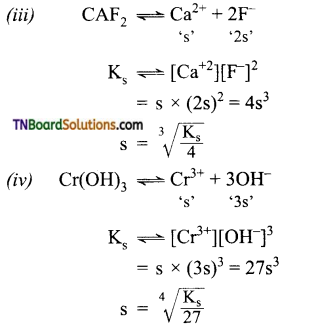Question 37.
Equal volumes of 0.002 M solutions of sodium iodate and cupric chlorate are mixed together. Will it lead to the precipitation of copper iodate? Ks for cupric iodate = 7.4 x 10-8.
Given, [NaIO3] = 0.002 M
[Cu(ClO3)2] = 0.002 M
∴ [IO3] = 0.002 M
[Cu+2] = 0.002 M
When equal volumes of these solutions are mixed, their concentrations will be halved.
i.e., [IO3] = 0.001 M
[Cu+2] = 0.001 M
Cu(IO3)2 ⇌ Cu+2 + 2IO3
Ks = [Cu+2][IO3]2
= 0.001 x (0.001)2 = 10-9
Since IP is less than solubility products precipitation will not occur.Question 38.
What is the minimum volume of water required to dissolve 1 gm of calcium sulfate at 298 K. The Ks for calcium sulfate is 9.1 x 10-6.
Let the solubility of CaSO4 at 298 K be ‘s’ mol L-1.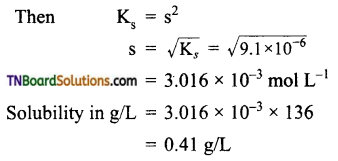Minimum volume of water required to dissolve
1 g of CaSO4 = $$\frac{1000}{0.41}$$
= 2439 mL = 2.439 L

Question 39.
The solubility product of BaS04 is 1.5 x 10-9 Find its solubility (i) in pure water and (ii) in 0.1M BaCl2, solution.
(i)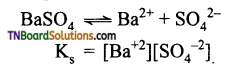Let ‘s’ be the solubility in mol L-1. Then
Ks = s2
(or) 1.5 x 107 = s2
s = $$\sqrt{1.5 \times 10^{-9}}$$
= 3.87 x 10-5 mol L7

(ii) Let (s’) be the solubility of BaSO4 in 0.1M BaCl2 solution.
Total [Ba2+] = [Ba2+] from BaSO4 from [Ba2+] from BaCl2.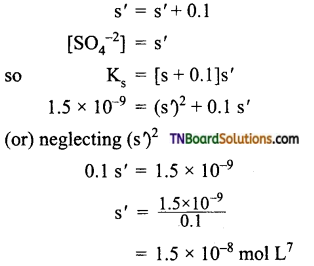Question 40.
Calculate the pH at which Mg(OH)2 begins to precipitate from a solution containing 0.10 Mg2+ ions.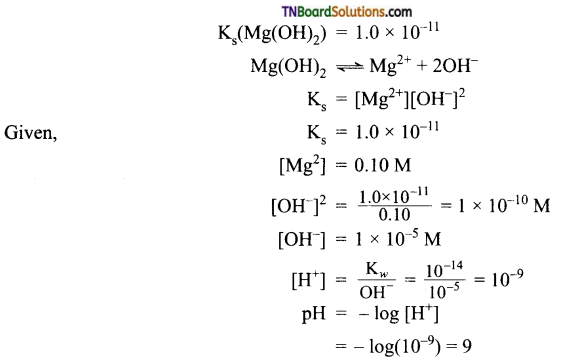1. What is the conjugate base of OH?
(a) O2
(b) H2O
(c) O
(d) O2-
(d)
Hint: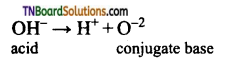2. C2H5ONa is …………. of C2H5OH.
(a) strong acid
(b) weak acid
(c) strong base
(d) weak base
(c)
Hint: C2H5OH is a strong base. a weak acid, C2H5O is a strong base3. Among the following, the one which can act as Bronsted acid as well as Bronsted base is:
(a) H3PO4
(b) AlCl3
(c) CH3COO
(d) H2O
(d)
Hint: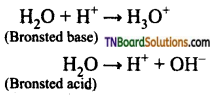4. At 25° C, the dissociation constant of a base, BOH is 1.0 x 10-12. The concentration of hydroxyl ions in 0.01M aqueous solution of the base would be:
(a) 1.0 x 10-6 mol lit-1
(b) 1.0 x 10-7 mol lit-1
(c) 2.0 x 10-6 mol lit-1
(d) 1.0 x 10-5 mol lit-1
(b)
Hint: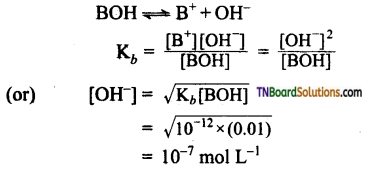5. Which of the following is the correct statement?
(a) HCO3 is the conjugate base of CO32-
(b) NH2 is the conjugate acid of NH3
(c) H2SO4 is the conjugate acid of HSO4
(d) NH3 is the conjugate base of NH2
(c)
Hint: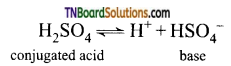6. Three reactions involving H2PO4 are given below: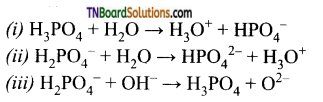In which of the above H2PO4 act as an acid?
(a) (iii) only
(b) (i) only
(c) (ii) only
(d) (i) and (ii)
(c)7. Which one of the following will decrease the pH of 50 ml of 0.01M hydrochloric acid?
(a) Addition of 50 ml of 0.01 M HCl
(b) Addition of 50 ml of 0.002 M HCl
(c) Addition of 150 ml of 0.002 M HCl
(d) Addition of 5 ml of 1 M HCl
(d)
Hint: For 0.001 M HCl, [H+] = 10-2M; pH = 2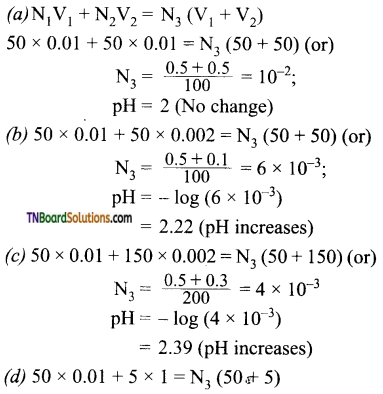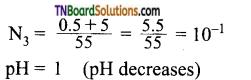8. If pKa for fluoride ion at 25° C, is 10.83, the ionisation constant of hydrofluoric acid at this temperature is:
(a) 1.74 x 10-5
(b) 3.52 x 10-3
(c) 6.75 x 10-4
(d) 5.38 x 10-2
(c)
Hint:
pKa + pKb = 14
pKa = 14 – 10.83 = 3.17
pKa= – log Ka = – log 3.17
log Ka= -3.17 = 4.83
(or) Ka= 6.76 x 10-4

9. The pH of a solution obtained by mixing 100 ml of a solution of pH = 3 with 400 ml of a solution of pH = 4 is:
(a) 3 – log 2.8
(b) 1 – log 2.8
(c) 4 – log 2.8
(d) 5 – log 2.8
(c)
Hint: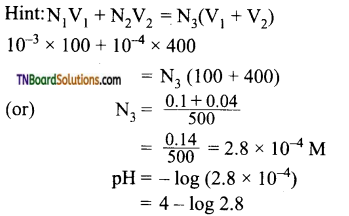10. The pH of 0.1M aqueous solution of a weak acid, HA is 3. What is its degree of dissociation?
(a) 1%
(b) 10%
(c) 50%
(d) 25%
(a)
Hint: HA ⇌ H+ + A
[H+] = Cα
0.1 x α = 10-3
α = 10-2 i.e., 1%

11. The pKa of acetic acid is 4.74. The concentration of acetic acid is 0.01 M. The pH of acetic acid is:
(a) 3.37
(b) 4.37
(c) 4.74
(d) 0.474
(a)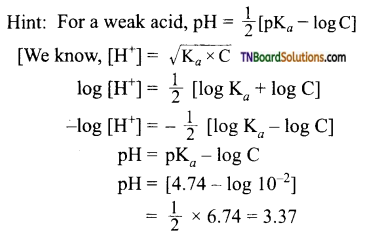12. How many times 1M CH3COOH solution should be diluted so that pH of the solution is doubled?
(a) 20 times
(b) 200 times
(c) 5.55 x 102 times
(d) 5.55 x 104 times
(d)
Hint: pH of a weak acid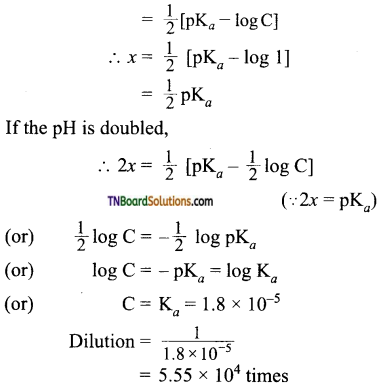13. 40 ml of 0.1M ammonia is mixed with 20 ml 0.1M HCl. What is the pH of the mixture? (pKb for ammonia is 4.74).
(a) 4.74
(b) 2.26
(c) 9.26
(d) 5.00
(c)
Hint: 40 ml of 0.1M NH3 solution
= 40 x 0.1 milli = 4 millimol
20 ml of 0.1 M HCl = 20 x 0.1 = 2 millimol
2 millimol of HCl neutralise 2 millimol of NH4OH, to form 2 millimol of NH4OH.
NH4OH left = 2 millimol
Total volume = 60 ml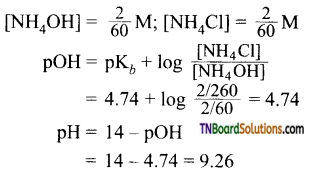14. The pH of a solution formed on mixing 20 ml of 0.05M H2SO4 with 5 ml of 0.45 M NaOH at 298 K is:
(a) 6
(b) 2
(c) 12
(d) 1
(c)
Hint:
2NaOH + H2SO4 → Na2SO4 + 2H2O
20 ml of 0.05M H2SO4
= 20 ml of 0.05 millimol
= 1.0 millimol

5 ml of 0.45M NaOH
= 5 x 0.45 millimol
= 2.25 millimol

2 millimol of NaOH will react with 1 millimol of H2SO4.
∴ NaOH left in solution = 0.5 millimol Volume of the solution = 25 ml
∴ [OH] = $$\frac{0.5}{25}$$ = 0.01 M = 10-2 M
[H+] = 10-12;
pH = 12

15. Which of the following salts will have the highest pH in water?
(a) KCl
(b) NaCl
(c) Na2CO3
(d) CuSO4
(c)
Hint: KCl and NaCl, being salts of strong acid and strong base do not get hydrolysed. Their aqueous solutions are neutral and hence their pH = 7.
Na2CO3 being a salt of weak acid and a strong base, gives NaOH as a product of hydrolysis. Hence, its aqueous solution is alkaline and has pH >7.
CuSO4 being a salt of strong acid and weak base, gives H2SO4, as a product of hydrolysis H2SO4 is a strong acid and its pH < 7.16. The H3O+ ion concentration of a solution of pH 6.58 is:
(a) antilog (- 6.58)
(c) antilog (- 5.58)
(a)
Hint: pH = – log [H3O+];
log [H3O+] = -pH = – 6.58
[H3O+] = antilog (-6.58)
= 2.63 x 10-7 g ion/lit

17. 30 CC of $$\frac{M}{4}$$ HCl, 20 CC of $$\frac{M}{2}$$ HNO3, and 40 CC of $$\frac{M}{4}$$ NaOH solutions are mixed and the volume is made upto 1 dm3. The pH of the resulting solution is:
(a) 2
(b) 1
(c) 3
(d) 8
(a)
Hint:
Total millimoles of H
= (30 x $$\frac{1}{3}$$) + (20 x $$\frac{1}{2}$$)
= 10 + 10 = 20
Total millimol of OH
= 40 x $$\frac{1}{4}$$ = 10
∴ H+ ions left after the neutralisation = 10 millimoles Volume of the solution
= 1 dm3
∴ Molarity of H+ ions
= $$\frac{10}{1000}$$ = 10-2M
pH = – log [H+]
= – log [10-2] = 2

18. The pH of 0.1 M solutions of the following salts increases in the order:
(a) NaCl < NH4Cl < NaCN < HCl
(b) HCl < NH4Cl < NaCl < NaCN
(c) NaCN < NH4Cl < NaCl < HCl
(d) HCl < NaCl < NaCN < NH4Cl
(b)
Hint: NaCl = neutral (pH = 7); NH4Cl = slightly acidic (pH < 7); NaCN = basic (pH > 7); HCl = strongly acidic (pH << 7).

19. A weak acid HX has the dissociation constant 1 x 10-5. It forms NaX on reaction with alkali. The degree of hydrolysis of 0.1 M solution of NaX is:
(a) 0.0001%
(b) 0.01 %
(c) 0.1%
(d) 0.15 %
(b)
Hint: Hydrolysis reaction is
X + H2O ⇌ HX + OH.
For a salt of weak acid with strong base,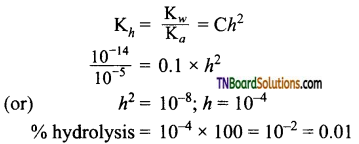20. Among the following hydroxides, the one which has the lowest value of Ks (solubility product) at ordinary temperature is:
(a) Mg(OH)2
(b) Ca(OH)2
(c) Ba(OH)2
(d) Be(OH)2
(d)
Hint: The solubility increases down the group due to an increase in the size of the ion and a decrease in lattice energy. Lower the solubility, lower is the Ks.

21. On adding 0.1M solution each of Ag+, Ba2+, Ca2+ ions in a Na2SO4 solution, the species first precipitated is (Ks(CaSO4) = 10-6, Ks(BaSO4) = 10-11, Ks(Ag2SO4) = 10-5:
(a) Ag2SO4
(b) BaSO4
(c) CaSO4
(d) All of these
(b)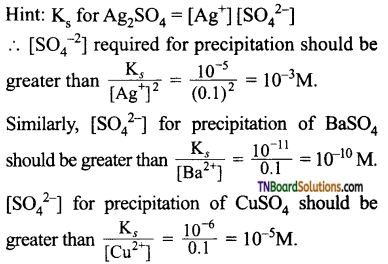The minimum [SO42-] concentration required for precipitation in for BaSO4.

22. The Ks of Ag2CrO4, AgCl, AgBr, and AgI are respectively 1.1 x 10-12, 1.8 x 10-6, 5.0 x 10-13 and 8.3 x 10-17. Which of the following salts will precipitate last of AgNO3 solution containing equal moles of NaCl, NaBr, Nal and Na2CrO4.
(a) AgBr
(b) Ag2CrO4
(c) AgI
(d) AgCl
(b)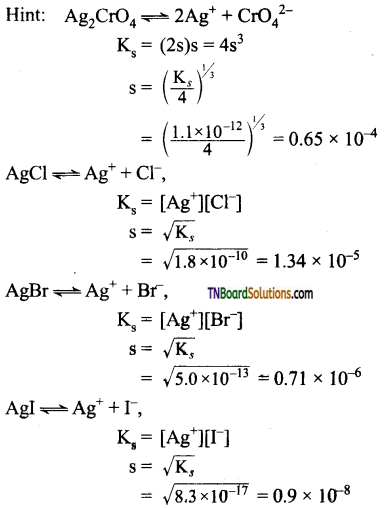As the solubility of Ag2CrO4 is highest, it will be precipitated last at all.23. The Ks of Ag2CrO4 is 1.1 x 10 12 at 298 K.
The solubility in mol per litre of Ag2CrO4 in 0.1M. AgNO3 solution is:
(a) 1.1 x 10-11
(b) 1.1 x 10-10
(c) 1.1 x 10-12
(d) 1.1 x 10-9
(b)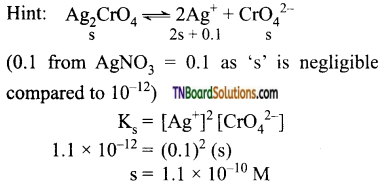24. Using Gibb’s free energy change, AG° = +63.3 kJ for the following reaction:
Ag2CO3 (s) ⇌ 2Ag+(aq) + CO32-(aq)
the Ks for Ag2CO3 in water at 25° C is (R = 8.314 JK-1 mol-1)
(a) 3.2 x 10-26
(b) 8.0 x 10-12
(c) 2.9 x 10-3
(d) 7.9 x 10-2
(b)
Hint: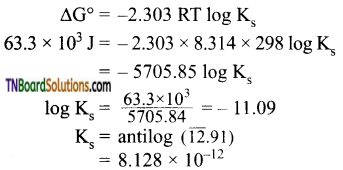25. A buffer solution is prepared in which the concentration of NH3 is 0.30M and the concentration of NH4+ is 0.20M. If the equilibrium constant, Kb for NH3 equals 1.8 x 10-5, what is the pH of the solution?
(a) 8.73
(b) 9.08
(c) 9.43
(d) 11.72
(b)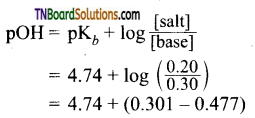= 4.74 – 0.176 = 4.56
pH = 14 – pOH = 14 – 4.56 = 9.44

26. What is the [H+] in mol/L of a solution that is 0.20M in CH3COONa and 0.10M in CH3COOH? Kb for CH3COOH = 1.8 x 10-5:
(a) 9.0 x 106
(b) 3.5 x 10-6
(b) 1.1 x 10-5
(d) 1.8 x 10-5
(a)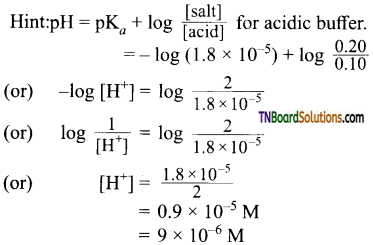27. In what volume ratio of NH4Cl and NH4OH solution (each 1M) should be mixed to get a buffer solution of pH, 9.80 (pKb for NH4OH is 4.74):
(a) 1 : 2.5
(b) 2.5 : 1
(c) 1 : 3.5
(d) 3.5 : 1
(c)
Hint:
Suppose ‘V1’ ml of 1M NH4Cl is mixed with ‘V2’ ml of 1M NH4OH solution:
V1 ml of 1M NH4Cl = V1 millimole
V2 ml of 1M NH4OH = V2 millimole
pH = 9.80, Hence pOH = 14 – 9.80 = 4.20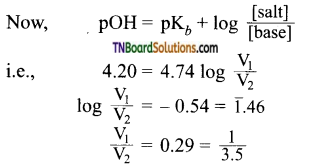28. Which one of the following pairs of solutions is not an acidic buffer?
(a) H2CO3 + Na2CO3
(b) H3PO4 + Na3PO4
(c) HClO4 + NaClO4
(d) CH3COOH + CH3COONa